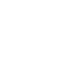Menu## Which is the maximum weight and size?

For mainland domestic service, the maximum weight of the volumes we carry is 30 kg and the total of the 3 dimensions should not exceed 1.60m and the largest measure cannot exceed 1.20m.

For international road service, the maximum weight of the volumes we carry is 31.5 kg and the total of the 3 dimensions must not exceed 3.00 m, calculated by the formula Length cm + 2 x (Width cm + Height cm). The maximum length is 1.75m. If the parcel exceeds the dimensions and is accepted by the DPD network, an additional fee of 26,00 € will be due. Volumetric weight is also applied to this service whenever it is higher than real weight, being calculated by the formula Width cm x Height cm x Length cm / 5000.

Volumetric Weight applies to the International Air and Islands service and whenever this is bigger than the actual weight of the order (according to the formula: Width cm x Height cm x Length cm / 4000 in the Islands, and Width cm x Height cm x Length cm / 5000 in international). Volumetric weight is applied to shipments to the Spanish Islands whenever it is bigger than the actual weight of the goods, using the formula Width cm x Height cm x Length cm / volumetric ratio. Volume ratios: Balearic Islands, Ceuta: 5000; Canary and Melilla: 3000.